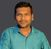Announcements
Applications are open for the 2024 Qlik Luminary Program. Apply by December 15 here.
cancel
Showing results for
Did you mean:Contributor III

## How to merge two values of one dimension via chart function

Hi experts,

I have one dimension with e.g three values

A,

B,

C,

Now I want to merge A and B in the chart (not via applymap in the script) to aggregrate their KPI value. I have to create a calculated dimension for this, right?. But my attempts all failed.

Any suggestions?

BR Ingo

Labels (3)

• ### merge values

1 Solution

Accepted SolutionsMVP

Option 1 : Recommended

``````Dim:
List
1
2 ];

Data:
Dim, Value
A, 10
B, 15
C, 30];

Dimension:
=pick(List,'A-B',Dim)

Expression:
=pick(List,sum({<Dim={'A','B'}>}Value),sum({<Dim-={'A','B'}>}Value))``````

Option 2: Without script

``````Data:
Dim, Value
A, 10
B, 15
C, 30];

Dimension:
=aggr(if(match(Dim,'A','B'),'A-B',only({<Dim-={'A','B'}>}Dim)),Dim)

Expression:
=if(not match(Dim,'A','B'),sum({<Dim-={'A','B'}>}Value),sum({<Dim={'A','B'}>}Value))``````

5 RepliesSpecialist

Hi,

can you explain using some examplesContributor III
Author

Hi,

my data looks like this:

Dim, Value

A, 10

B, 20

C, 30

Now it should look like:

Dim, Value

A (represents A and B), 30 (A+B)

C, 30

Does this help?

BR

IngoContributor III
Author

Hi guys,

come on!

Nobody could help?

BR

IngoMVP

Option 1 : Recommended

``````Dim:
List
1
2 ];

Data:
Dim, Value
A, 10
B, 15
C, 30];

Dimension:
=pick(List,'A-B',Dim)

Expression:
=pick(List,sum({<Dim={'A','B'}>}Value),sum({<Dim-={'A','B'}>}Value))``````

Option 2: Without script

``````Data:
Dim, Value
A, 10
B, 15
C, 30];

Dimension:
=aggr(if(match(Dim,'A','B'),'A-B',only({<Dim-={'A','B'}>}Dim)),Dim)

Expression:
=if(not match(Dim,'A','B'),sum({<Dim-={'A','B'}>}Value),sum({<Dim={'A','B'}>}Value))``````Contributor III
Author

Many thanks!Tags
Community Browser